# How to Alphabetize Comma-Separated Strings in Google Sheets?

Google Sheets is an amazing tool that helps us perform incredible tasks. One of the incredible tasks that we can perform in Google Sheets is to arrange the strings in alphabetical order. To arrange the strings in alphabetical order, we will have to use various Google Sheets functions such as SPLIT, TRANSPOSE, and JOIN.
With the help of Google Sheets Tips, we will learn how to alphabetize the comma-separated strings. Read further to find out more.

## Alphabetizing Comma-Separated Strings in Google Sheets

Consider the following dataset in Google Sheets: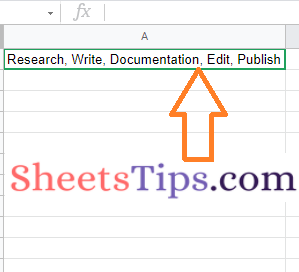Now we need to arrange the following dataset in an alphabetical manner. The step-by-step procedure to get this done is explained in the following section.

### How to Sort Alphabetically in Google Sheets and Keep Rows Together?

The simple steps to sorting the data in alphabetical order are as follows:

### Step 1: The Split Function Is Used in the First Step

• We have the list of strings arranged in Cell A1.
• Now move to the cell where you want to alphabetize the string.
• Enter the formula =SPLIT(A1, “,”) here and hit the “Return” key.
• Now the data is being split as shown in the image below.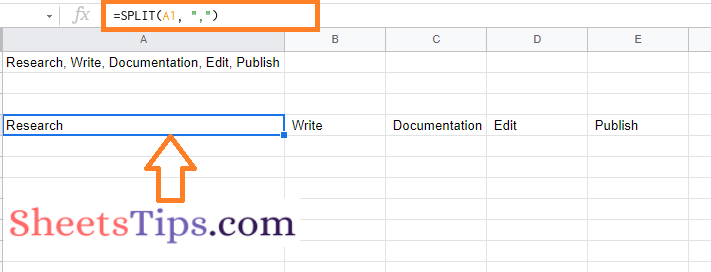### Step 2: Making Use of the Transpose Function

Now we will have to use the Transpose function to change the row directly into the column direction. We need to use the transpose function in the row since we will have to use the SORT function. The steps for using the TRANSPOSE function in Google Sheets are explained below.

• Move to the cell where you have used the SPLIT function.
• Now modify the SPLIT function with the TRANSPOSE function and enter the formula as =TRANSPOSE (SPLIT (A1, “,”)).
• Press the “Enter” button and now you will see the results.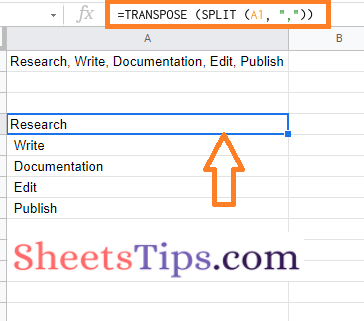### Step 3: Applying the SORT Formula

Now the next step is to SORT data using the SORT formula. To SORT data using the SORT function is as follows:

• Move to the cell where you have applied the SPLIT and TRANSPOSE formulas.
• Now modify the formula with the SORT function and enter the formula as =SORT(TRANSPOSE (SPLIT (A1, “,”)))
• Press the “Enter” formula and you will see the results as shown below.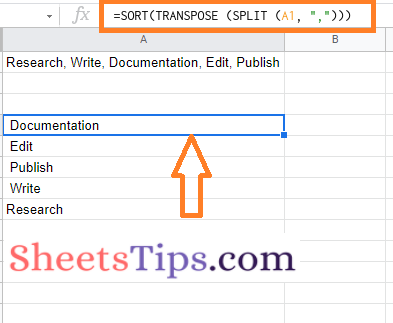### Step 4: Using the JOIN Function

Now we come to the final step, with the help of which we can sort the data in alphabetical order. The steps to using the join function to arrange the data in alphabetical order are as follows:

• Move to the cell where you have used the SORT Formula.
• Now modify the formula using the JOIN function. The formula which needs to be adjusted is as follows: “=JOIN(“,”,SORT(TRANSPOSE(SPLIT(A1,“,”))))
• Press the “Enter” button and you will see the results.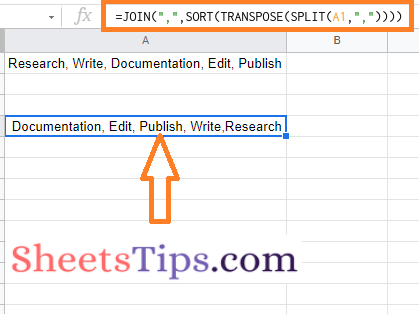That’s it. Our data is being sorted in alphabetical order, as shown in the image below.